• 访问像素值
• 用指针扫描图像
• 使用迭代器扫描图像
• 编写有效的图像扫描循环
• 使用邻居访问扫描图像
• 执行简单的图像算术
• 定义兴趣区域

# 简介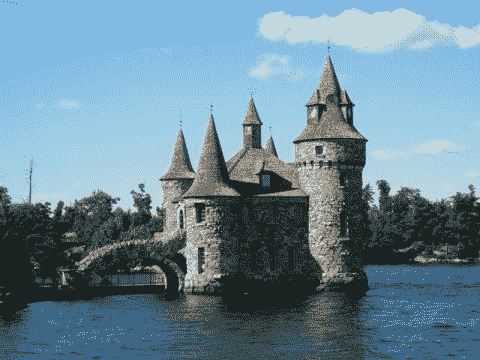# 访问像素值

## 操作步骤

void salt(cv::Mat &image, int n) {

for (int k=0; k<n; k++) {

// rand() is the MFC random number generator
// try qrand() with Qt
int i= rand()%image.cols;
int j= rand()%image.rows;

if (image.channels() == 1) { // gray-level image

image.at<uchar>(j,i)= 255;

} else if (image.channels() == 3) { // color image

image.at<cv::Vec3b>(j,i)= 255;
image.at<cv::Vec3b>(j,i)= 255;
image.at<cv::Vec3b>(j,i)= 255;
}
}
}


   // open the image

// call function to add noise
salt(image,3000);

// display image
cv::namedWindow("Image");
cv::imshow("Image",image);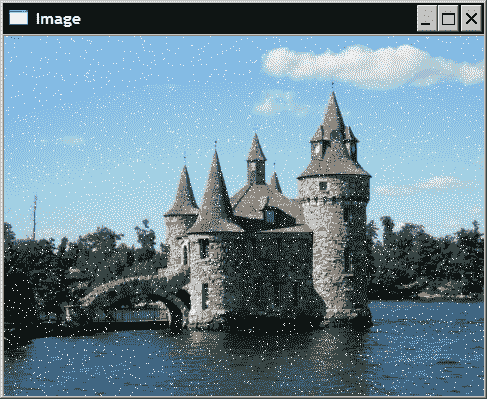## 工作原理

cv::Mat包括几种访问图像不同属性的方法。 公共成员变量colsrows为您提供图像中的列数和行数。 对于元素访问，cv::Mat具有方法at(int y, int x)。 但是，必须在编译时知道方法返回的类型，并且由于cv::Mat可以保存任何类型的元素，因此程序员需要指定期望的返回类型。 这就是at方法已被实现为模板方法的原因。 因此，在调用它时，必须指定图像元素类型，如下所示：

         image.at<uchar>(j,i)= 255;


         image.at<cv::Vec3b>(j,i)[channel]= value;


2 元素和 4 元素向量（cv::Vec2bcv::Vec4b）以及其他元素类型也存在类似的向量类型。 在此后一种情况下，最后一个字母由shortsintifloatfdoubled替换。 所有这些类型都是使用模板类cv::Vec<T,N>定义的，其中T是类型，N是向量元素的数量。

## 更多

   cv::Mat_<uchar> im2= image; // im2 refers to image


## 另见

“编写高效的图像扫描循环”秘籍可讨论此方法的效率。

# 用指针扫描图像

## 操作步骤

void colorReduce(cv::Mat &image, int div=64);


void colorReduce(cv::Mat &image, int div=64) {

int nl= image.rows; // number of lines
// total number of elements per line
int nc= image.cols * image.channels();

for (int j=0; j<nl; j++) {

// get the address of row j
uchar* data= image.ptr<uchar>(j);

for (int i=0; i<nc; i++) {

// process each pixel ---------------------

data[i]=    data[i]/div*div + div/2;

// end of pixel processing ----------------

} // end of line
}
}


   // read the image
// process the image
colorReduce(image);
// display the image
cv::namedWindow("Image");
cv::imshow("Image",image);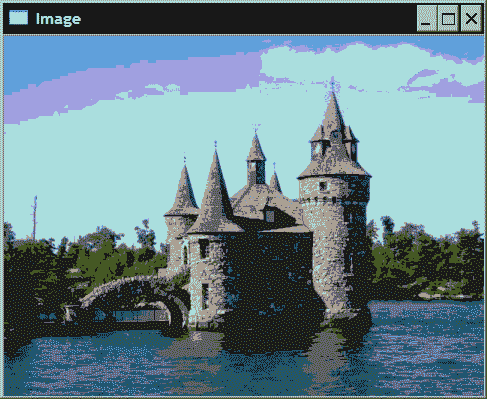## 工作原理

     int nc= image.cols * image.channels();


        uchar* data= image.ptr<uchar>(j);


        *data++= *data/div*div + div2;


## 更多

### 其他颜色缩减秘籍

     data[i]= data[i]/div*div + div/2;


     data[i]=    data[i] – data[i]%div + div/2;


     // mask used to round the pixel value


     data[i]=    (data[i]&mask) + div/2;


### 具有输入和输出参数

   // read the image
// clone the image
cv::Mat imageClone= image.clone();
// process the clone
// orginal image remains untouched
colorReduce(imageClone);
// display the image result
cv::namedWindow("Image Result");
cv::imshow("Image Result",imageClone);


void colorReduce(const cv::Mat &image, // input image
cv::Mat &result,      // output image
int div=64);


colorReduce(image,image);


cv::Mat result;
colorReduce(image,result);


     result.create(image.rows,image.cols,image.type());


     for (int j=0; j<nl; j++) {

// get the addresses of input and output row j
const uchar* data_in= image.ptr<uchar>(j);
uchar* data_out= result.ptr<uchar>(j);

for (int i=0; i<nc; i++) {

// process each pixel ---------------------

data_out[i]= data_in[i]/div*div + div/2;

// end of pixel processing ----------------

} // end of line


### 高效扫描连续图像

void colorReduce(cv::Mat &image, int div=64) {

int nl= image.rows; // number of lines
int nc= image.cols * image.channels();

if (image.isContinuous())
{
nc= nc*nl;
nl= 1;  // it is now a 1D array
}

// this loop is executed only once
// in case of continuous images
for (int j=0; j<nl; j++) {

uchar* data= image.ptr<uchar>(j);

for (int i=0; i<nc; i++) {

// process each pixel ---------------------

data[i]= data[i]/div*div + div/2;

// end of pixel processing ----------------

} // end of line
}
}


     if (image.isContinuous())
{
image.reshape(1,            // new number of channels
image.cols*image.rows) ; // new number of rows
}

int nl= image.rows; // number of lines
int nc= image.cols * image.channels();


### 低级指针算法

cv::Mat类中，图像数据包含在unsigned char的存储块中。 该存储块第一个元素的地址由data属性给定，该属性返回一个无符号的char指针。 因此，要在图像的开头开始循环，您可以编写：

uchar *data= image.data;


data+= image.step;  // next line


step方法为您提供一行中的字节总数（包括填充的像素）。 通常，您可以按以下方式获取行j和列i的像素地址：

// address of pixel at (j,i) that is &image.at(j,i)
data= image.data+j*image.step+i*image.elemSize();


## 另见

“编写高效的图像扫描循环”秘籍用于讨论此处介绍的扫描方法的效率。

# 使用迭代器扫描图像

## 操作步骤

     cv::MatIterator_<cv::Vec3b> it;


     cv::Mat_<cv::Vec3b>::iterator it;


void colorReduce(cv::Mat &image, int div=64) {

// obtain iterator at initial position
cv::Mat_<cv::Vec3b>::iterator it=
image.begin<cv::Vec3b>();
// obtain end position
cv::Mat_<cv::Vec3b>::iterator itend=
image.end<cv::Vec3b>();

// loop over all pixels
for ( ; it!= itend; ++it) {

// process each pixel ---------------------

(*it)= (*it)/div*div + div/2;
(*it)= (*it)/div*div + div/2;
(*it)= (*it)/div*div + div/2;

// end of pixel processing ----------------
}
}


## 工作原理

     while (it!= itend) {

// process each pixel ---------------------
...

// end of pixel processing ----------------

++it;
}


operator++是用于移至下一个元素的那个。 您还可以指定更大的步长。 例如，it+=10将每 10 个像素处理一次。

     cv::MatConstIterator_<cv::Vec3b> it;


     cv::Mat_<cv::Vec3b>::const_iterator it;


## 更多

     cv::Mat_<cv::Vec3b> cimage= image;
cv::Mat_<cv::Vec3b>::iterator it= cimage.begin();
cv::Mat_<cv::Vec3b>::iterator itend= cimage.end();


## 另见

“编写高效的图像扫描循环”秘籍讨论了扫描图像时迭代器的效率。

# 编写有效的图像扫描循环

## 操作步骤

double duration;
duration = static_cast<double>(cv::getTickCount());

colorReduce(image); // the function to be tested

duration = static_cast<double>(cv::getTickCount())-duration;
duration /= cv::getTickFrequency(); // the elapsed time in ms


colorReduce函数的测试中，我们还实现了使用at方法进行像素访问的函数版本。 然后，此实现的主循环将读为：

      for (int j=0; j<nl; j++) {
for (int i=0; i<nc; i++) {

// process each pixel ---------------------

image.at<cv::Vec3b>(j,i)=
image.at<cv::Vec3b>(j,i)/div*div + div/2;
image.at<cv::Vec3b>(j,i)=
image.at<cv::Vec3b>(j,i)/div*div + div/2;
image.at<cv::Vec3b>(j,i)=
image.at<cv::Vec3b>(j,i)/div*div + div/2;

// end of pixel processing ----------------

} // end of line
}


## 工作原理

data[i]= data[i]/div*div + div/2 ; 37ms
*data++= *data/div*div + div/2; 37ms
*data++= v - v%div + div/2; 52ms
*data++= *data&mask + div/2; 35ms
colorReduce(input, output); 44ms
i<image.cols*image.channels(); 65ms
MatIterator 67ms
.at(j,i) 80ms
3-channel loop 29ms

 int nc= image.cols * image.channels();
...
for (int i=0; i<nc; i++) {


      for (int i=0; i<image.cols * image.channels(); i++) {


void colorReduce(cv::Mat &image, int div=64) {

int nl= image.rows; // number of lines
int nc= image.cols ; // number of columns

// is it a continous image?
if (image.isContinuous())  {
nc= nc*nl;
nl= 1;  // it is now a 1D array
}

int n= static_cast<int>(
log(static_cast<double>(div))/log(2.0));
// mask used to round the pixel value

// for all pixels
for (int j=0; j<nl; j++) {

// pointer to first column of line j
uchar* data= image.ptr<uchar>(j);

for (int i=0; i<nc; i++) {

// process each pixel ---------------------

// end of pixel processing ----------------

} // end of line
}
}


# 使用邻居访问扫描图像

## 准备

sharpened_pixel= 5*current-left-right-up-down;


## 操作步骤

void sharpen(const cv::Mat &image, cv::Mat &result) {

// allocate if necessary
result.create(image.size(), image.type());

for (int j= 1; j<image.rows-1; j++) { // for all rows
// (except first and last)

const uchar* previous=
image.ptr<const uchar>(j-1); // previous row
const uchar* current=
image.ptr<const uchar>(j);     // current row
const uchar* next=
image.ptr<const uchar>(j+1); // next row

uchar* output= result.ptr<uchar>(j); // output row

for (int i=1; i<image.cols-1; i++) {

*output++= cv::saturate_cast<uchar>(
5*current[i]-current[i-1]
-current[i+1]-previous[i]-next[i]);
}
}

// Set the unprocess pixels to 0
result.row(0).setTo(cv::Scalar(0));
result.row(result.rows-1).setTo(cv::Scalar(0));
result.col(0).setTo(cv::Scalar(0));
result.col(result.cols-1).setTo(cv::Scalar(0));
}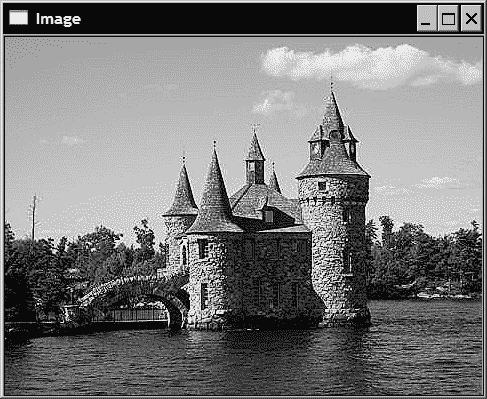## 工作原理

   result.row(0).setTo(cv::Scalar(0));


## 更多

0 -1 0
-1 5 -1
0 -1 0

void sharpen2D(const cv::Mat &image, cv::Mat &result) {

// Construct kernel (all entries initialized to 0)
cv::Mat kernel(3,3,CV_32F,cv::Scalar(0));
// assigns kernel values
kernel.at<float>(1,1)= 5.0;
kernel.at<float>(0,1)= -1.0;
kernel.at<float>(2,1)= -1.0;
kernel.at<float>(1,0)= -1.0;
kernel.at<float>(1,2)= -1.0;

//filter the image
cv::filter2D(image,result,image.depth(),kernel);
}


# 执行简单的图像运算

## 准备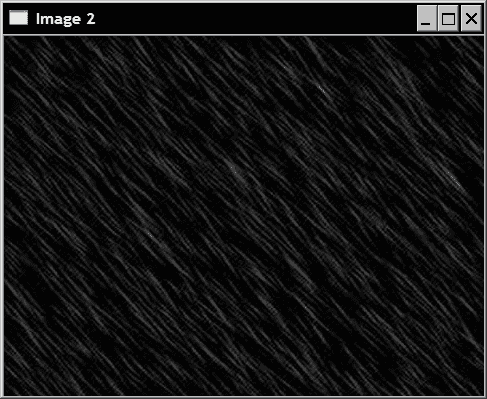## 操作步骤

   cv::addWeighted(image1,0.7,image2,0.9,0.,result);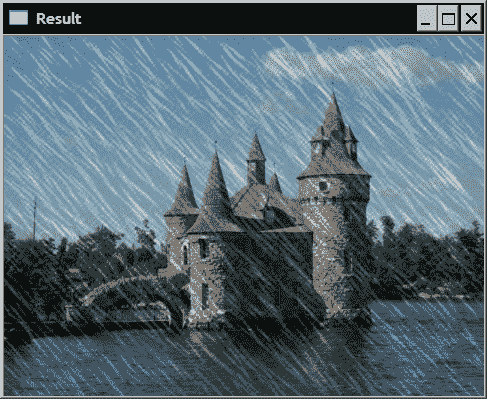## 工作原理

   // c[i]= a[i]+b[i];
// c[i]= a[i]+k;
// c[i]= k1*a+k2*b[i]+k3;
// c[i]= k*a+b[i];


   // if (mask[i]) c[i]= a[i]+b[i];


## 更多

### 重载的图像运算符

result= 0.7*image1+0.9*image2;


     image=(image&cv::Scalar(mask,mask,mask))
+cv::Scalar(div/2,div/2,div/2);


cv::Scalar的使用是由于我们正在处理彩色图像。 执行与在“编写高效的图像扫描循环”秘籍中所做的相同测试，我们获得89ms的执行时间。 这主要是因为如所写，该表达式需要调用两个函数，按位与和标量和（而不是在一个图像循环内执行完整的操作）。 即使生成的代码并非始终是最佳的，使用图像运算符也使代码如此简单，并且程序员如此高效，以至于在大多数情况下都应考虑使用它们。

### 分割图像通道

   // create vector of 3 images
std::vector<cv::Mat> planes;
// split 1 3-channel image into 3 1-channel images
cv::split(image1,planes);
planes+= image2;
// merge the 3 1-channel images into 1 3-channel image
cv::merge(planes,result);


cv::merge函数执行双重操作，即从三个 1 通道图像创建彩色图像。

# 定义兴趣区域

## 准备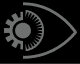## 操作步骤

   // define image ROI
cv::Mat imageROI;
imageROI= image(cv::Rect(385,270,logo.cols,logo.rows));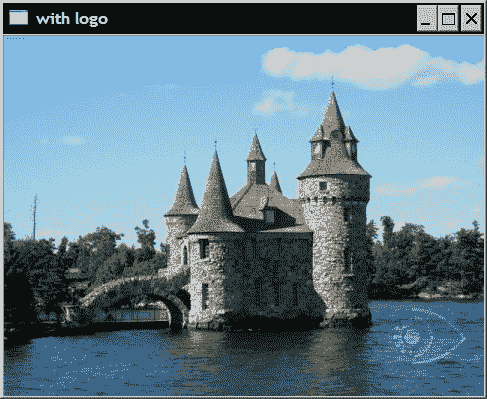// define ROI
imageROI= image(cv::Rect(385,270,logo.cols,logo.rows));

// copy to ROI with mask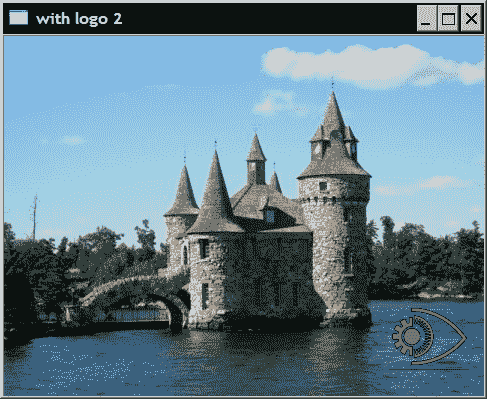## 工作原理

cv::Mat imageROI= image(cv::Range(270,270+logo.rows),
cv::Range(385,385+logo.cols))


cv::Matoperator()返回另一个cv::Mat实例，该实例随后可用于子序列调用中。 ROI 的任何变换都会影响相应区域中的原始图像，因为图像和 ROI 共享相同的图像数据。 由于 ROI 的定义不会复制数据，因此无论 ROI 的大小如何，它都将在固定时间内执行。

cv::Mat imageROI= image.rowRange(start,end) ;


cv::Mat imageROI= image.colRange(start,end) ;